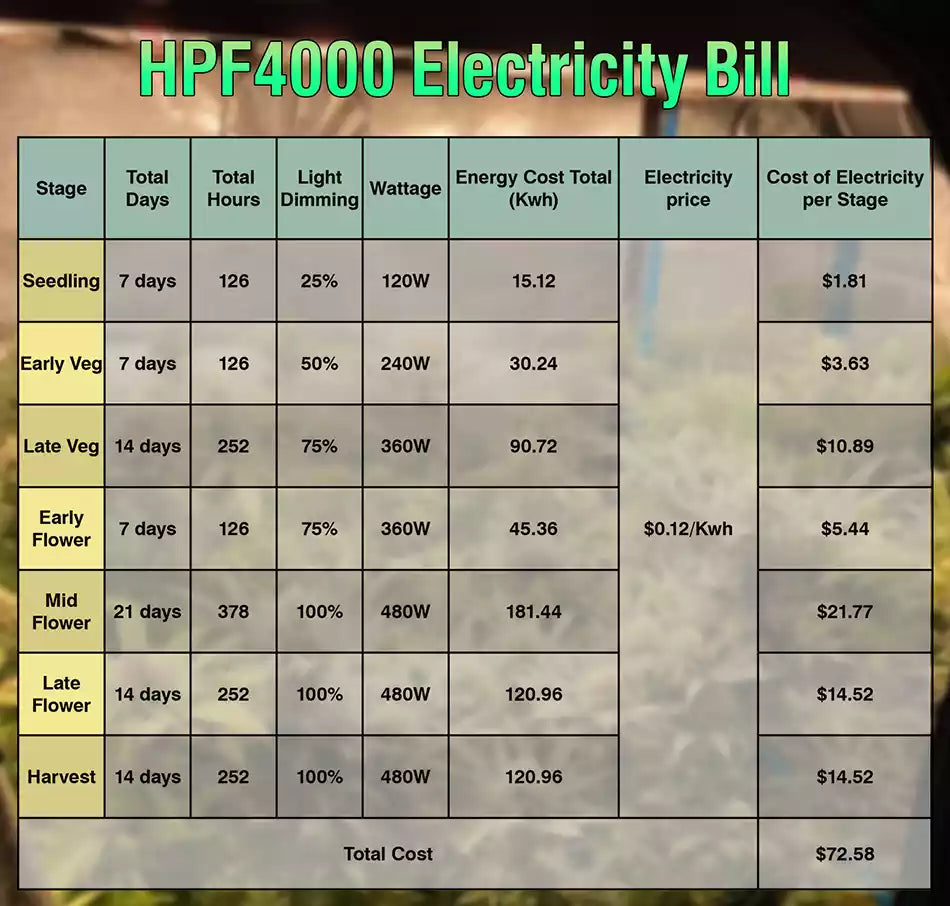Introduction to Grow light spectrum, PAR, PPF, PPFD & PPE Reading How much electricity bill it cost in a full cycle(Autoflower) under HPF4000? 2 minutes Next The production schedule about led grow light of HYPHOTONFLUX (part 4)
##### How much electricity bill it cost in a full cycle(Autoflower) under HPF4000?
✿ We have so many growers use our HPF4000, but do you know how much electricity bill it cost in a full cycle? Let me figure it out for you.
✿ Since growers have different timeline on Photoperiod cannabis, so we take autoflower as an example to calculate.
✿ Strain: Autoflower
✿ Light: HPF4000(Max. 480watt)
✿ Light Schedules: 18/6
✿ To calculate how much electricity an LED grow light uses isn't as difficult as you would imagine. You just need the wattage of the light and the number of hours during the day that the light is running.
✿ The LEDs will generally have how many kilowatts they draw. Once you identify how many kilowatts, they draw you need to convert that into kilowatts per hour or kwh. To do this, divide the draw by 1000. So the HPF4000 has a power draw of 0.48 kwh when dimming it to 100% and 0.24 kwh when dimming it to 50%.
✿ Now, you just calculate how long the lights are running, let's say18 hours per day times the 0.48 kwh. That gives us consumption of 8.64-kilowatt hours. The next thing you need to know is the electricity rate that you're charged.✿ As I know that the average price of electricity in the US is 12.83 cents per kilowatt-hour, which equals \$0.1283 per kwh, so it's easy to count the total cost.
✿ In the table Above I listed the kwh you consume each stage and electricity bill, finally I got the total cost 72.58\$. Sounds unbelievable, right? But it is the truth and that's why LED is more and more popular, especially for commercial growers, LED save a lot cost in electricity bill for them
If you are still running hps, I suggest you try LED from now on.